##### Statistics For Dummies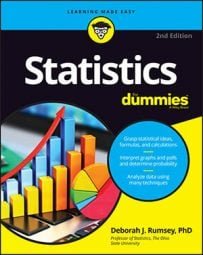If you are working from a large statistical sample, then solving problems using the binomial distribution might seem daunting. However, there's actually a very easy way to approximate the binomial distribution, as shown in this article.

Here's an example: suppose you flip a fair coin 100 times and you let X equal the number of heads. What's the probability that X is greater than 60?

In a situation like this where n is large, the calculations can get unwieldy and the binomial table runs out of numbers. So if there's no technology available (like when taking an exam), what can you do to find a binomial probability? Turns out, if n is large enough, you can use the normal distribution to find a very close approximate answer with a lot less work.

But what do we mean by n being "large enough"? To determine whether n is large enough to use what statisticians call the normal approximation to the binomial, both of the following conditions must hold: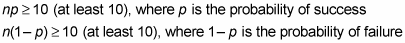To find the normal approximation to the binomial distribution when n is large, use the following steps:

1. Verify whether n is large enough to use the normal approximation by checking the two appropriate conditions.

For the above coin-flipping question, the conditions are met because n p = 100 ∗ 0.50 = 50, and n ∗ (1 – p) = 100 ∗ (1 – 0.50) = 50, both of which are at least 10. So go ahead with the normal approximation.

2. Translate the problem into a probability statement about X.

In this example, you need to find p(X > 60).

3. Standardize the x-value to a z-value, using the z-formula: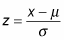For the mean of the normal distribution, use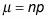(the mean of the binomial), and for the standard deviation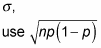(the standard deviation of the binomial).

So, in the coin-flipping example, you have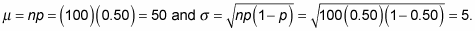Then put these values into the z-formula to get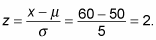To solve the problem, you need to find p(Z > 2).

On an exam, you won't see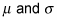in the problem when you have a binomial distribution. However, you know the formulas that allow you to calculate both of them using n and p (both of which will be given in the problem). Just remember you have to do that extra step to calculate theneeded for the z-formula. You can now proceed as you usually would for any normal distribution.

4. Look up the z-score on the Z-table and find its corresponding probability.

• a. Find the row of the table corresponding to the leading digit (one digit) and first digit after the decimal point (the tenths digit).

• b. Find the column corresponding to the second digit after the decimal point (the hundredths digit).

• c. Intersect the row and column from Steps (a) and (b).

Continuing the example, from the z-value of 2.0, you get a corresponding probability of 0.9772 from the Z-table.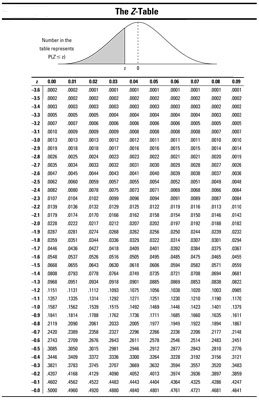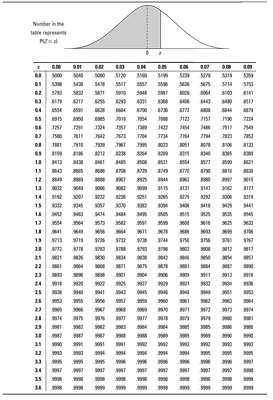5. Select one of the following.

• a. If you need a "less-than" probability — that is, p(X < a) — you're done.

• b. If you want a "greater-than" probability — that is, p(X > b) — take one minus the result from Step 4.

Remember, this example is looking for a greater-than probability ("What's the probability that X — the number of flips — is greater than 60?"). Plugging in the result from Step 4, you find p(Z > 2.00) = 1 – 0.9772 = 0.0228. So the probability of getting more than 60 heads in 100 flips of a coin is only about 2.28 percent. (In other words, don't bet on it.)

• c. If you need a "between-two-values" probability — that is, p(a < X < b) — do Steps 1–4 for b (the larger of the two values) and again for a (the smaller of the two values), and subtract the results.

When using the normal approximation to find a binomial probability, your answer is an approximation (not exact) — be sure to state that. Also show that you checked both necessary conditions for using the normal approximation.